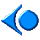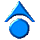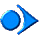Unfortunately, before you learn how to split your program into multiple execution threads, all programs you wrote contain a single execution thread. The following diagram shows an example. Suppose we have a program of two parts, Part A and Part B. After Part A finishes its computation, we use some cout statements to print out a large amount of output. As we all know, when a program prints, the control is transfered to a function in C++'s library and the execution of that program is essentially suspended, shown in dashed line in the diagram, until the printing completes. Once this (i.e., printing) is done, the execution of the program resumes and starts the computation of Part B. Is there anything wrong with this? No, we are used to it and we are trained to do programming in this way ever since CS101. However, is this way of programming good enough in terms of efficiency? It depends; but, in many situations, it is not good enough.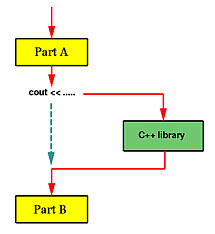If Part B must use some data generated by Part A, then Part B perhaps has to be executed after the output of cout completes. On the other hand, in many situations, Part A and Part B are independent of each other, or one may slightly rewrite both parts so that they do not depend on each other. In this case, Part B does not have to wait until cout completes. In fact, this is the key point! Therefore, before the execution of Part A, the program can be split into two execution threads, one for Part A and the other for Part B. See the diagram below. In this way, both execution threads share the CPU and all resources allocated to the program. Moreover, while Part A is performing the output which causes Part A to wait, Part B can take the CPU and executes. As a result, this version is more efficient than the previous one. Moreover, in a system with more than one CPUs, it is possible that the system will run both Part A and Part B at the same time, one on each CPU.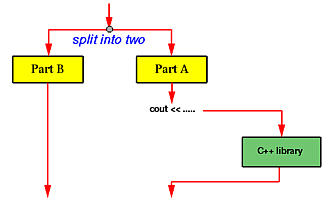In real programming practice, a program may use an execution thread for handling keyboard/mouse input, a second execution thread for handling screen updates, and a number of other threads for carrying out various computation tasks.

### Example: Quicksort

The quicksort algorithm consists of two steps in each recursion. First, the partition step divides the input array segment into two segments such that all elements in the left segment is smaller or equal to all elements in the right segment. Second, the sorting step simply sorts the left segment and the right segment. After these two steps complete, the input segment is sorted. While it is not so obvious if the partition step can have multiple execution threads, one can split the execution of the sorting step into two, one for sorting the left segment while the other for sorting the right one. This is shown in the diagram below: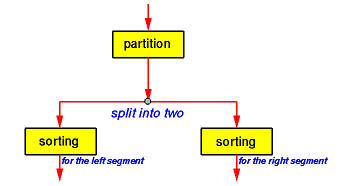### Example: Merging

Consider another simple problem. Suppose we have two arrays a[ ] and b[ ] of n elements each. For simplicity, we assume that all of these 2n elements are different. Our job is to merge these two arrays into a sorted one. Everyone who took a data structures course knows how to do it; however, let us look at the same problem from a different angle.

Take an element from array a, say a[i]. We know that it is larger than i-1 elements of a. If we can figure out how many elements of b that are smaller than a[i], we will be able to know the exact location of a[i] in the sorted array. This is illustrated in the following diagram: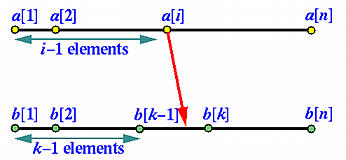With a slightly modified binary search, we can easily determine the location of a[i] in array b. There are only three possibilities:

1. a[i] is less than b: In this case, a[i] is larger than i-1 elements in a and smaller than all elements in b. Therefore, a[i] should be in position i of the sorted array.
2. a[i] is larger than b[n]: In this case, a[i] is larger than i-1 elements in a and n elements in b. Therefore, a[i] should be in position i+n of the sorted array.
3. a[i] is between b[k-1] and b[k]. In this case, a[i] is larger than i-1 elements in a and k-1 elements in b. Therefore, a[i] should be in position i+k-1 of the sorted array.
After the main program reads in both arrays, it can split itself into 2n execution threads, each of which handles an element in a or in b. Each of these execution threads determines its position in the merged array and writes the values into the corresponding location. After this, we will have a merged array! Thus, we use 2n threads, each of which takes O(log2(n)) comparisons to get the job done. In the conventional serial case, we use one execution thread which uses O(n) comparisons to merge the arrays.

### Example: Matrix Multiplication

Another interesting application is the multiplication of two matrices. Suppose we have two matrices Am×k (m rows and k columns) and Bk×n (k rows and n columns) and want to compute the product of A and B into a matrix C of m rows and n columns. The entry of C on row i and column j is the sum of the products of the corresponding elements on row i of matrix A and column j of matrix B as shown below: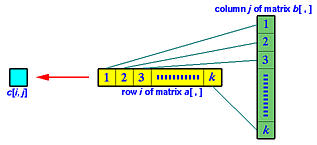How can we use multiple execution threads to solve this problem? We notice that the computation of Ci,j is independent of the computation of any other entries of matrix C. Because of this, after matrices A and B are read in, the main program can split m×n execution threads, one for each entry of matrix C. Each of these execution threads computes the products of the corresponding elements, sums them up, and stores the result into matrix C.

It requires k multiplications to compute a single entry of matrix C. Since there are m×n entries in C, the program with only one thread uses m×n×k multiplications. On the other hand, in the above scheme, each thread uses k multiplications and there are m×n threads. If we have only one CPU, the multiple execution threads version may not be as efficient as the single execution thread one; however, if there are more than one CPUs, each of these CPUs may be assigned to a number of execution threads and the execution efficiency is higher. In the extreme case in which we have m×n CPUs to use, because all execution threads run at the same time, it only takes the time to compute one entry to complete the whole matrix multiplication. Thus, it is m×n times more efficient than the single execution thread version.